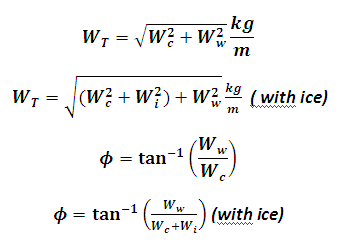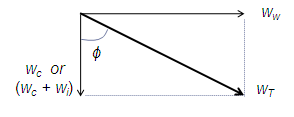# Angle of loading of conductor of a transmission line Calculator

This Calctown Calculator calculates the angle of loading of a conductor of a transmission line.

kg/m
kg/m
kg/m

#### Result

kg/m
radClick here to view image

where

WT = equivalent weight

Ww = wind loading

Wc = weight of conductor

Wi = weight of ice layer on conductor

φ = angle of loading